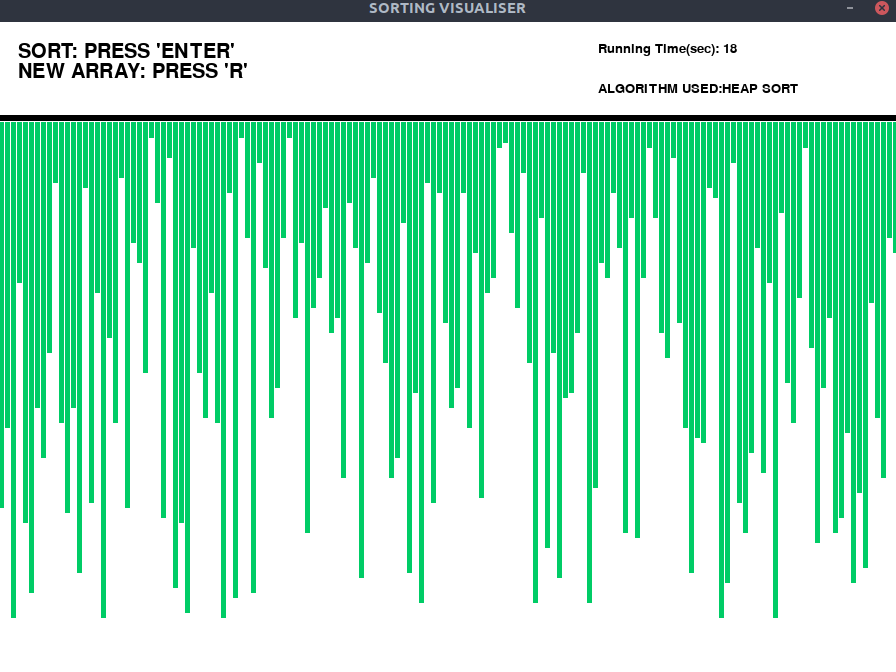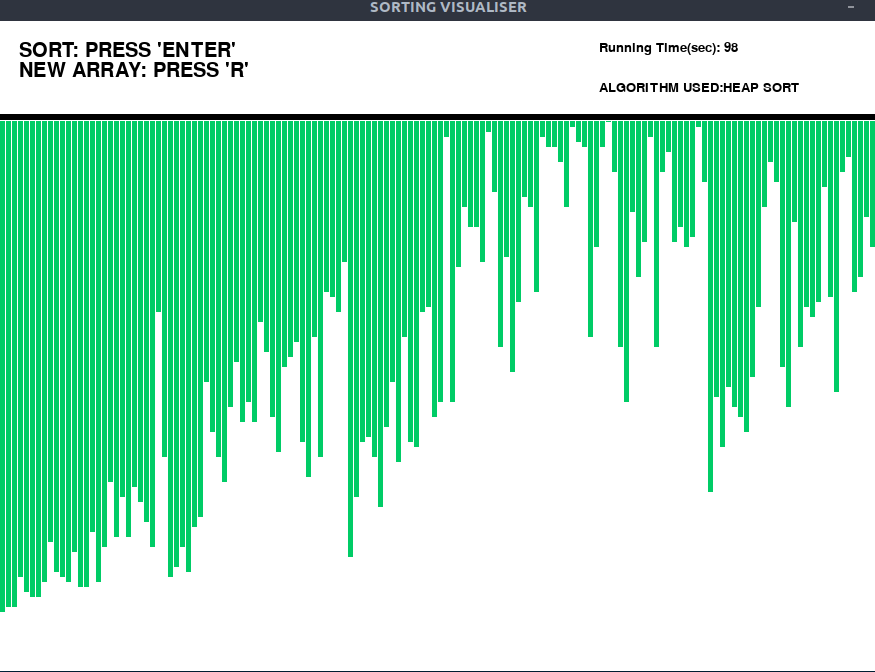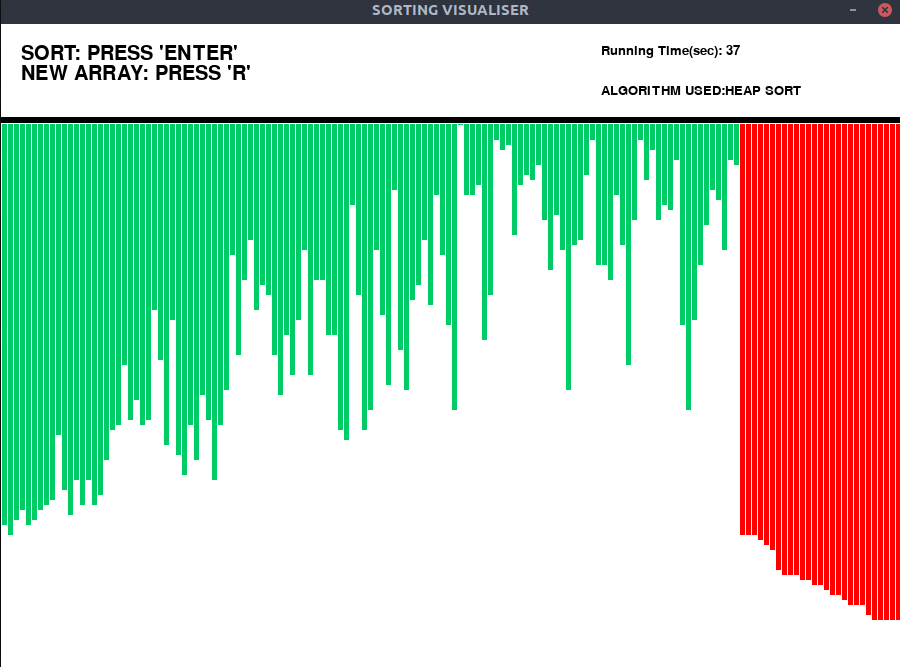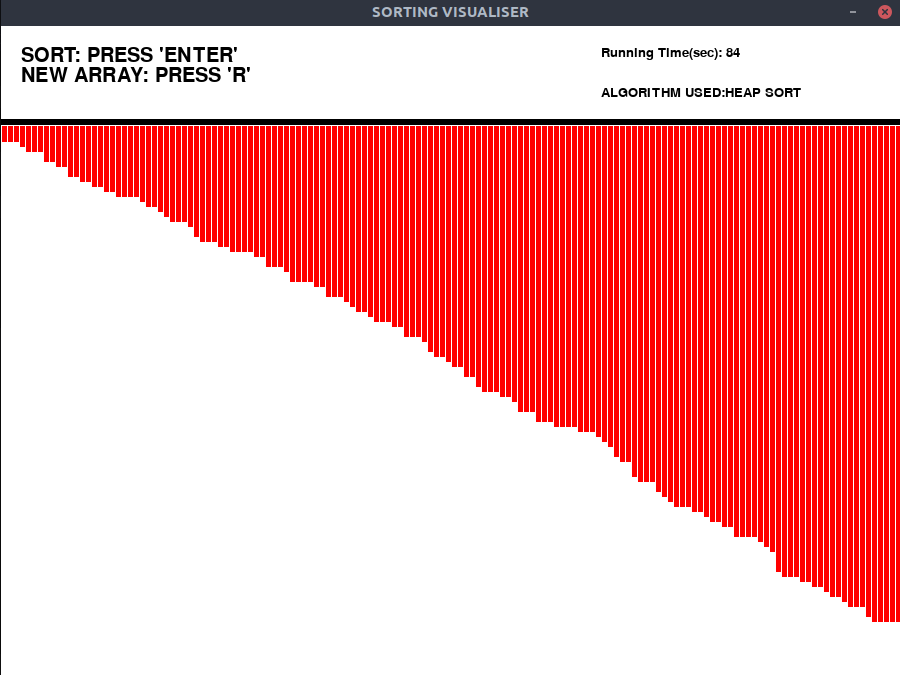# Sorting algorithm visualization : Heap Sort

An algorithm like Heap sort can be understood easily by visualizing. In this article, a program that visualizes the Heap Sort Algorithm has been implemented.

The Graphical User Interface(GUI) is implemented in Python using pygame library.

### Approach:

Generate random array and fill the pygame window with bars. Bars are straight vertical lines, which represents array elements.

• Set all bars to green color (unsorted).
• Heapify the array to perform sorting.
• After Heapify, large bars are at the beginning followed by smaller bars.
• Use pygame.time.delay() to slow down the algorithm, so that we can see the sorting process.
• Implement a timer to see how the algorithm performs.
• The actions are performed using ‘pygame.event.get()’ method, which stores all the events which user performs, such as start, reset.
• Blue color is used to highlight bars that are involved in sorting at a particular time.
• Orange color highlights the bars sorted.

### Observations:

We can clearly see from the Heap Sort visualization, that Heap Sort is very fast compared to other sorting algorithms like Insertion sort or Selection sort and similar in speed with Merge sort.

### Examples:

#### Input:

Press “Enter” key to Perform Visualization.

Press “R” key to generate new array.

#### Initial:#### After heapification of array:#### Sorting:#### Final:Please make sure to install the pygame library before running the below program.

Below is the implementation of the above visualizer:

## Python

 `# Python implementation of the ` `# Sorting visualiser: Heap Sort ` ` `  `# Imports ` `import` `pygame ` `import` `random ` `import` `time ` `pygame.font.init() ` `startTime ``=` `time.time() ` ` `  `# Total window ` `screen ``=` `pygame.display.set_mode( ` `    ``(``900``, ``650``) ` `) ` ` `  `# Title and Icon ` `pygame.display.set_caption( ` `    ``"SORTING VISUALISER"` `) ` ` `  `# Uncomment below lines for setting ` `# up the icon for the visuliser ` `# img = pygame.image.load('sorticon.png') ` `# pygame.display.set_icon(img) ` ` `  `# Boolean variable to run ` `# the program in while loop ` `run ``=` `True` ` `  `# Window size and some initials ` `width ``=` `900` `length ``=` `600` `array ``=` `[``0``]``*``151` `arr_clr ``=` `[(``0``, ``204``, ``102``)]``*``151` `clr_ind ``=` `0` `clr ``=` `[(``0``, ``204``, ``102``), (``255``, ``0``, ``0``), ` `       ``(``0``, ``0``, ``153``), (``255``, ``102``, ``0``)] ` `fnt ``=` `pygame.font.SysFont(``"comicsans"``, ``30``) ` `fnt1 ``=` `pygame.font.SysFont(``"comicsans"``, ``20``) ` ` `  `# Function to generate new Array ` `def` `generate_arr(): ` `    ``for` `i ``in` `range``(``1``, ``151``): ` `        ``arr_clr[i] ``=` `clr[``0``] ` `        ``array[i] ``=` `random.randrange(``1``, ``100``) ` ` `  ` `  `# Initially generate a array ` `generate_arr() ` ` `  `# Function to refill the ` `# updates on the window ` `def` `refill(): ` `    ``screen.fill((``255``, ``255``, ``255``)) ` `    ``draw() ` `    ``pygame.display.update() ` `    ``pygame.time.delay(``10``) ` ` `  ` `  `# Sorting Algorithm: Heap Sort ` `def` `heapSort(array): ` `    ``n ``=` `len``(array) ` `    ``for` `i ``in` `range``(n``/``/``2``-``1``, ``-``1``, ``-``1``): ` `        ``pygame.event.pump() ` `        ``heapify(array, i, n) ` `    ``for` `i ``in` `range``(n``-``1``, ``0``, ``-``1``): ` `        ``array[i], array[``0``] ``=` `array[``0``], array[i] ` `        ``arr_clr[i] ``=` `clr[``1``] ` `        ``refill() ` `        ``heapify(array, ``0``, i) ` ` `  ` `  `def` `heapify(array, root, size): ` `    ``left ``=` `root ``*` `2` `+` `1` `    ``right ``=` `root ``*` `2` `+` `2` `    ``largest ``=` `root ` `    ``if` `left < size ``and` `array[left] > array[largest]: ` `        ``largest ``=` `left ` `    ``if` `right < size ``and` `array[right] > array[largest]: ` `        ``largest ``=` `right ` `    ``if` `largest !``=` `root: ` `        ``arr_clr[largest] ``=` `clr[``2``] ` `        ``arr_clr[root] ``=` `clr[``2``] ` `        ``array[largest],\ ` `        ``array[root] ``=` `array[root],\ ` `        ``array[largest] ` `        ``refill() ` `        ``arr_clr[largest] ``=` `clr[``0``] ` `        ``arr_clr[root] ``=` `clr[``0``] ` `        ``heapify(array, largest, size) ` `        ``refill() ` ` `  `# Function to Draw the array values ` `def` `draw(): ` ` `  `    ``# Text should be rendered ` `    ``txt ``=` `fnt.render(``"SORT: PRESS 'ENTER'"``, ` `                     ``1``, (``0``, ``0``, ``0``)) ` `    ``# Position where text is placed ` `    ``screen.blit(txt, (``20``, ``20``)) ` `    ``txt1 ``=` `fnt.render(``"NEW ARRAY: PRESS 'R'"``, ` `                      ``1``, (``0``, ``0``, ``0``)) ` `    ``screen.blit(txt1, (``20``, ``40``)) ` `    ``txt2 ``=` `fnt1.render(``"ALGORITHM USED:"` `+` `                       ``"HEAP SORT"``, ``1``, (``0``, ``0``, ``0``)) ` `    ``screen.blit(txt2, (``600``, ``60``)) ` `    ``text3 ``=` `fnt1.render(``"Running Time(sec): "` `+` `                        ``str``(``int``(time.time() ``-` `startTime)), ` `                        ``1``, (``0``, ``0``, ``0``)) ` `    ``screen.blit(text3, (``600``, ``20``)) ` `    ``element_width ``=` `(width``-``150``)``/``/``150` `    ``boundry_arr ``=` `900` `/` `150` `    ``boundry_grp ``=` `550` `/` `100` `    ``pygame.draw.line(screen, (``0``, ``0``, ``0``), (``0``, ``95``), ` `                     ``(``900``, ``95``), ``6``) ` ` `  `    ``# Drawing the array values as lines ` `    ``for` `i ``in` `range``(``1``, ``151``): ` `        ``pygame.draw.line(screen, arr_clr[i], ` `                         ``(boundry_arr ``*` `i``-``3``, ``100``), ` `                         ``(boundry_arr ``*` `i``-``3``, ` `                          ``array[i]``*``boundry_grp ``+` `100``),\ ` `                         ``element_width) ` ` `  ` `  `# Program should be run ` `# continuously to keep the window open ` `while` `run: ` `    ``# background ` `    ``screen.fill((``255``, ``255``, ``255``)) ` ` `  `    ``# Event handler stores all event ` `    ``for` `event ``in` `pygame.event.get(): ` ` `  `        ``# If we click Close button in window ` `        ``if` `event.``type` `=``=` `pygame.QUIT: ` `            ``run ``=` `False` `        ``if` `event.``type` `=``=` `pygame.KEYDOWN: ` `            ``if` `event.key ``=``=` `pygame.K_r: ` `                ``generate_arr() ` `            ``if` `event.key ``=``=` `pygame.K_RETURN: ` `                ``heapSort(array) ` `    ``draw() ` `    ``pygame.display.update() ` ` `  `pygame.quit() `

Attention reader! Don’t stop learning now. Get hold of all the important DSA concepts with the DSA Self Paced Course at a student-friendly price and become industry ready.

My Personal Notes arrow_drop_upCheck out this Author's contributed articles.

If you like GeeksforGeeks and would like to contribute, you can also write an article using contribute.geeksforgeeks.org or mail your article to contribute@geeksforgeeks.org. See your article appearing on the GeeksforGeeks main page and help other Geeks.

Please Improve this article if you find anything incorrect by clicking on the "Improve Article" button below.

Practice Tags :

Be the First to upvote.

Please write to us at contribute@geeksforgeeks.org to report any issue with the above content.